# Determine your size for kitchen sinks

Flex P-trap can be used in all situations under the sink as a p-trap and as a connecting tube between parts. Because of this, you have to determine the size (length of tube) or cut the tube yourself. When replacing a regular p-trap with the Flex P-trap, you have to measure the length of the existing p-trap outside of the U shape and add one inch for safety. If you are looking from outside inside of cabinet, you have the right length of Flex P-trap noted with R and left length notated with L . The tolerance for measurement is ±0.5 inches.

1. First, you have to choose one position from Fig.18 - Fig.27
2.  Second, you have to measure dimension A, B, C and JK
3.  Third, you choose length R and L from bottom drawing or bottom measurement

CP-trap—connecting tube with p-trap

SP-trap—connecting S-trap with p-trap

Measurement Fig.18--Fig.22

Our converter formula was specially designed to figure out length of the p-trap a lot easier. Measure the distance JK in space and the length of Flex p-trap will be:

L=JK+10”

J—end of outlet tee, elbow etc.

K—bottom of drain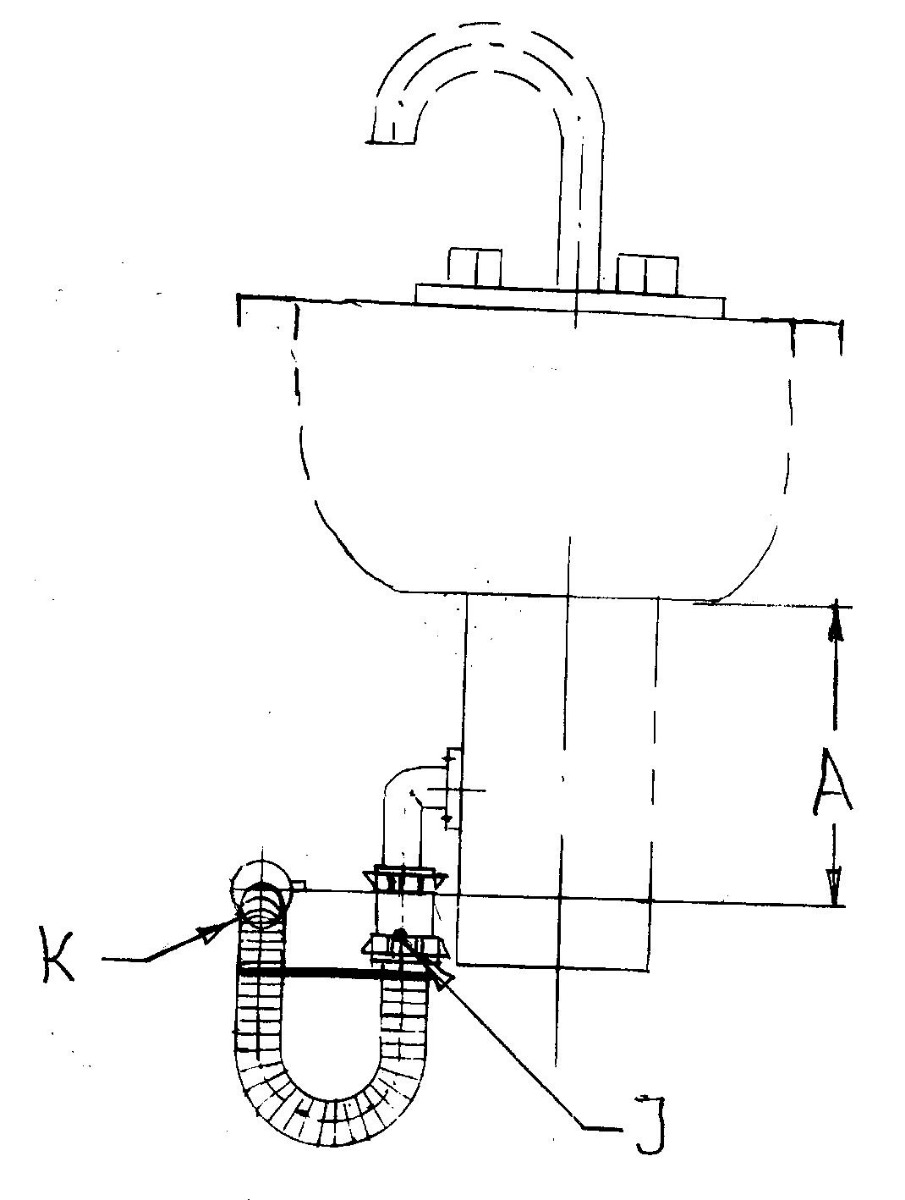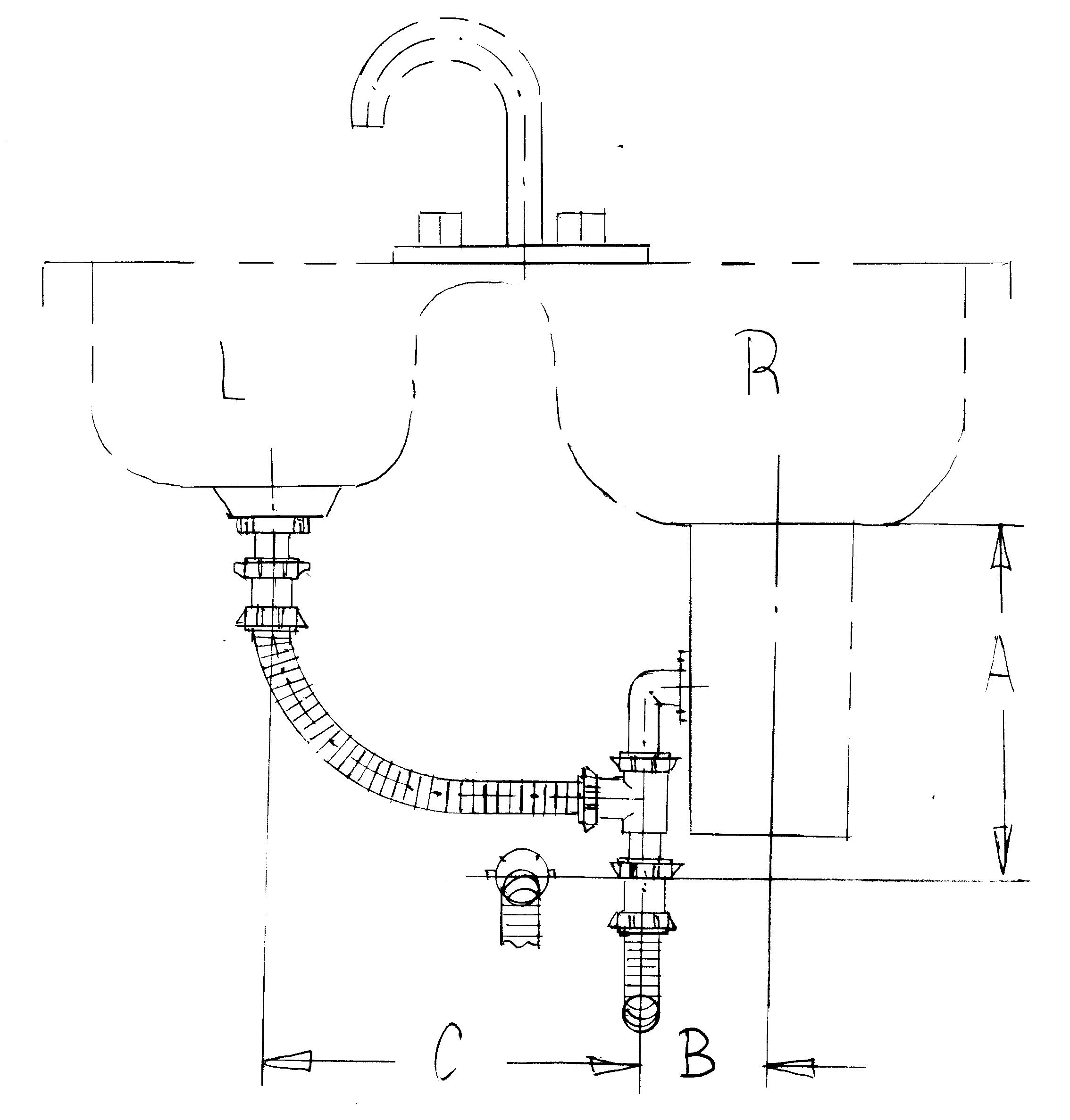Fig.18  L=JK+10 Fig.19 (video) L=C+B  R= 24 inches or CP22 CP=connecting tube with p-trap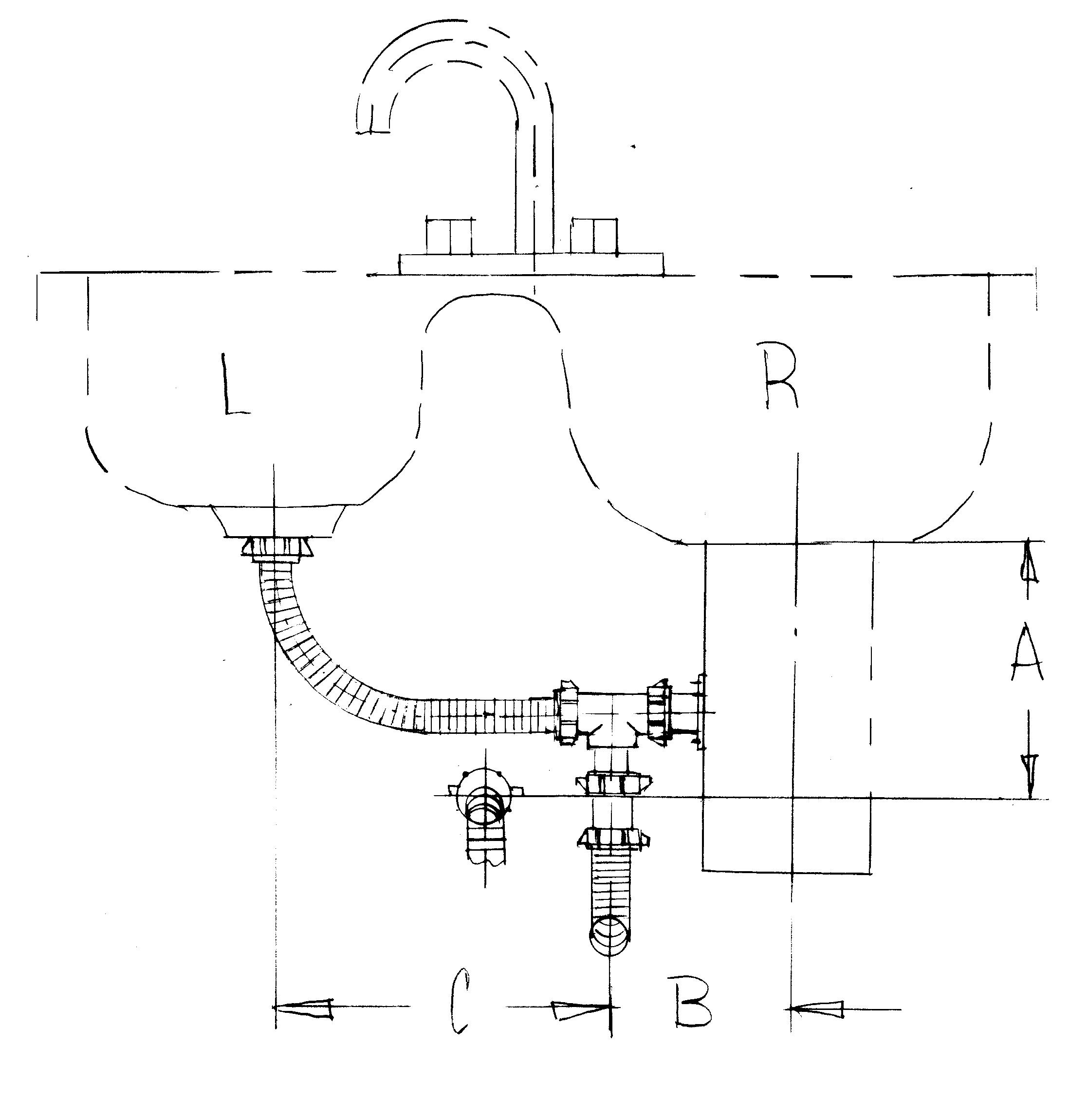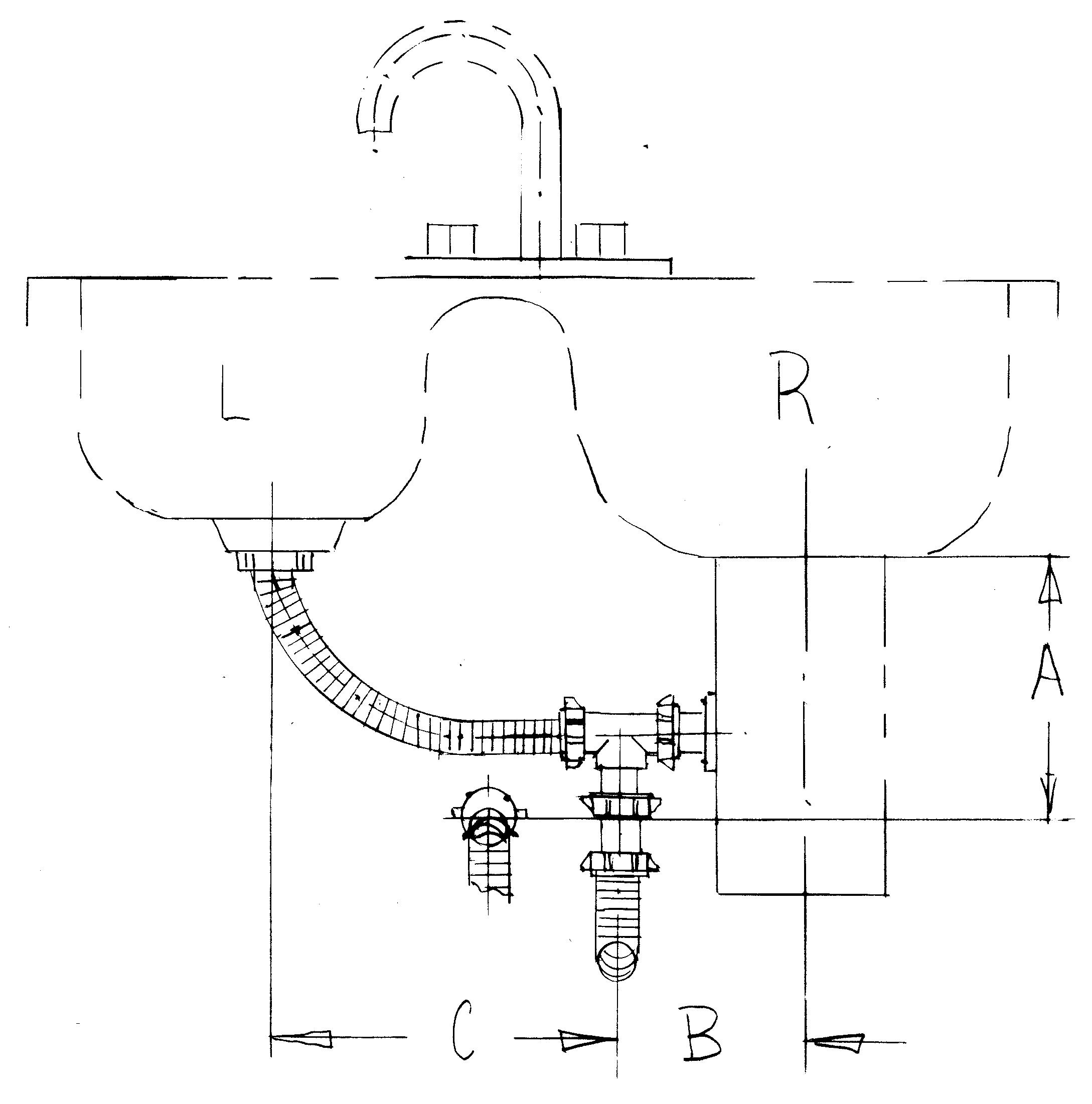Fig.20A (video)  L=C+B  R=24 inches or CP22 Fig.20B (video)  L=C+B  R=24 inches or CP22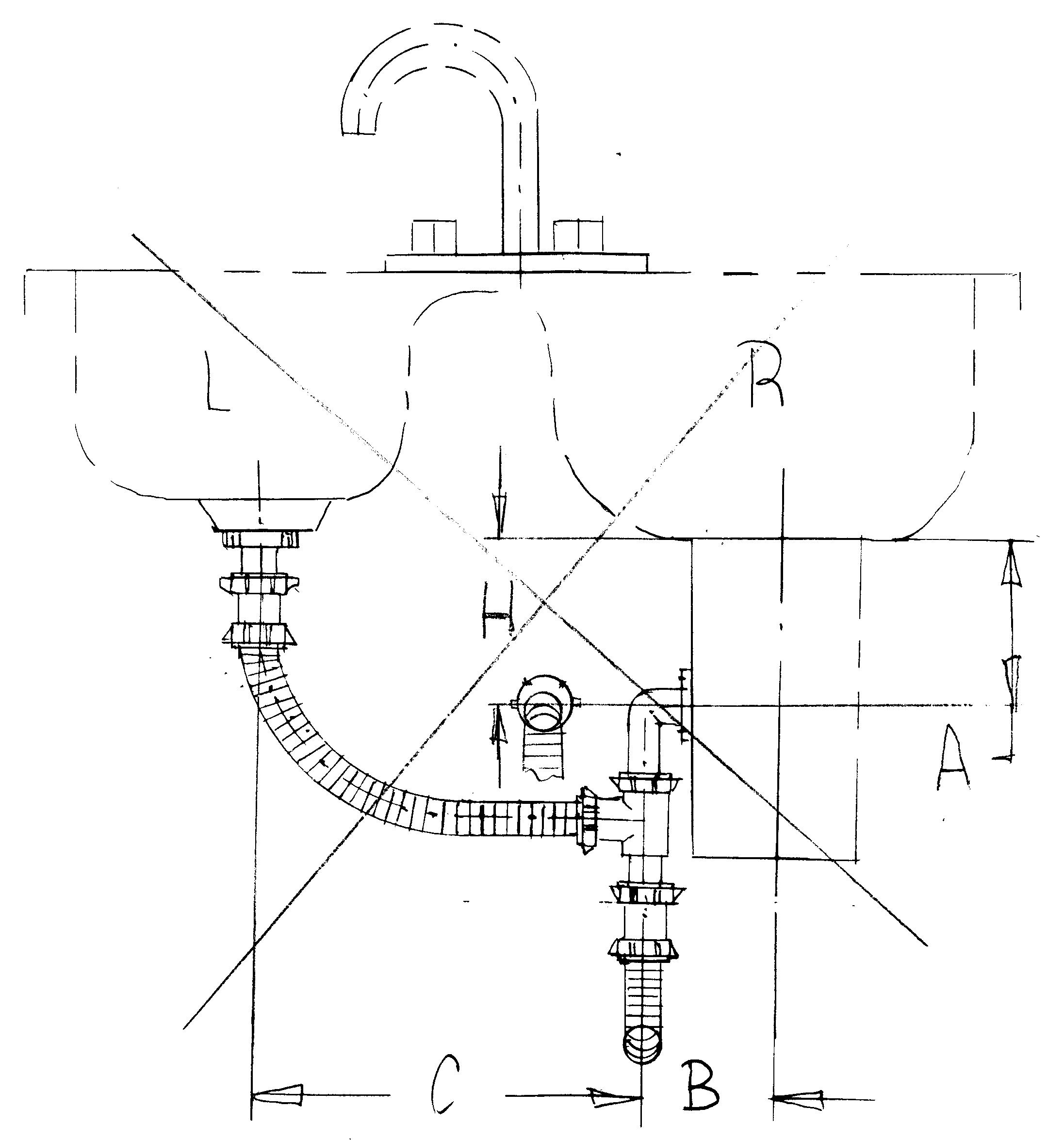Fig.20 C

If the drain it is on same level with disposal drain A=H, some water from the sink will remain in the garbage disposal; because of this, it's recommended that drain’s wall is lower than the garbage disposal drain. See the Fig.21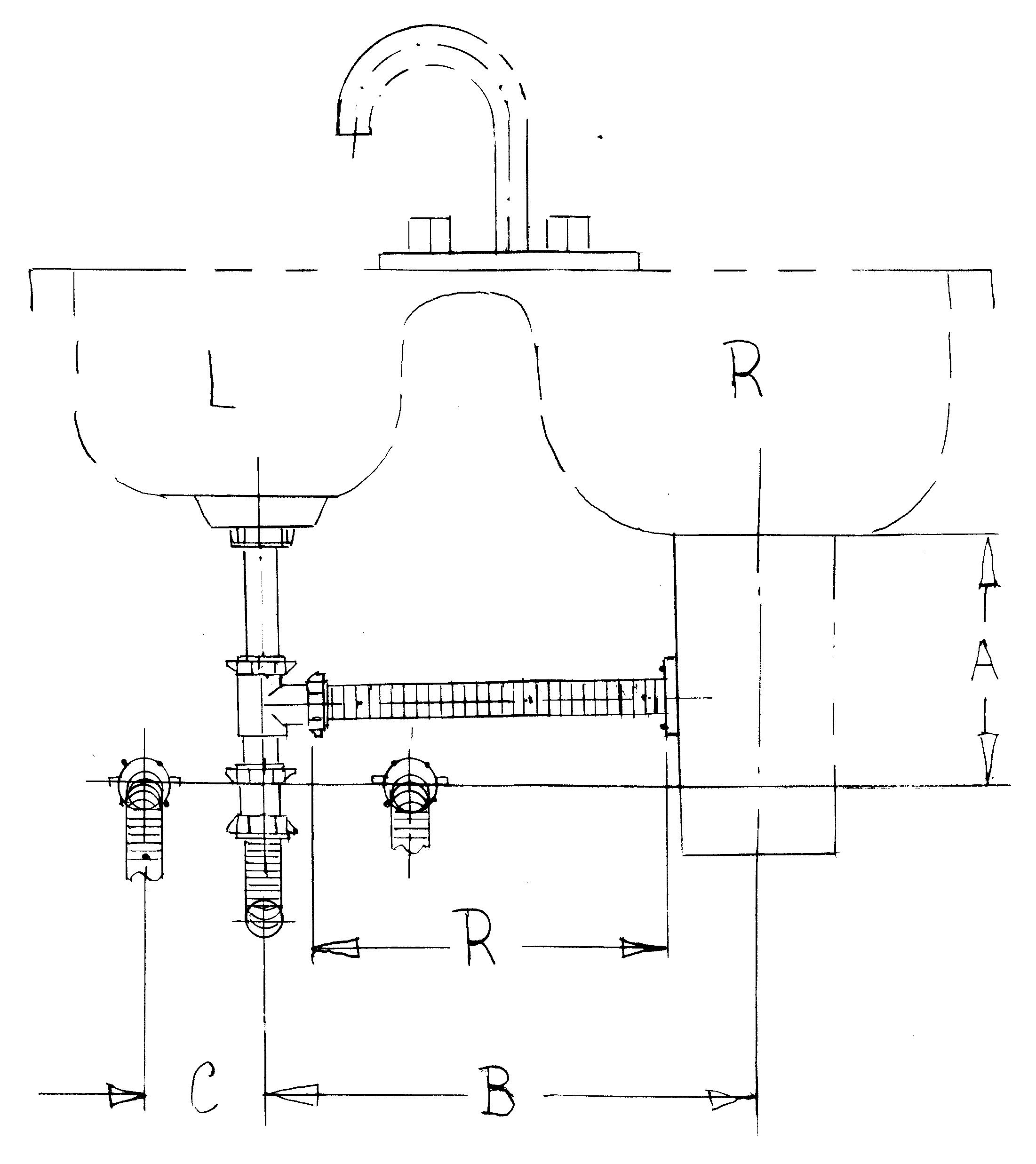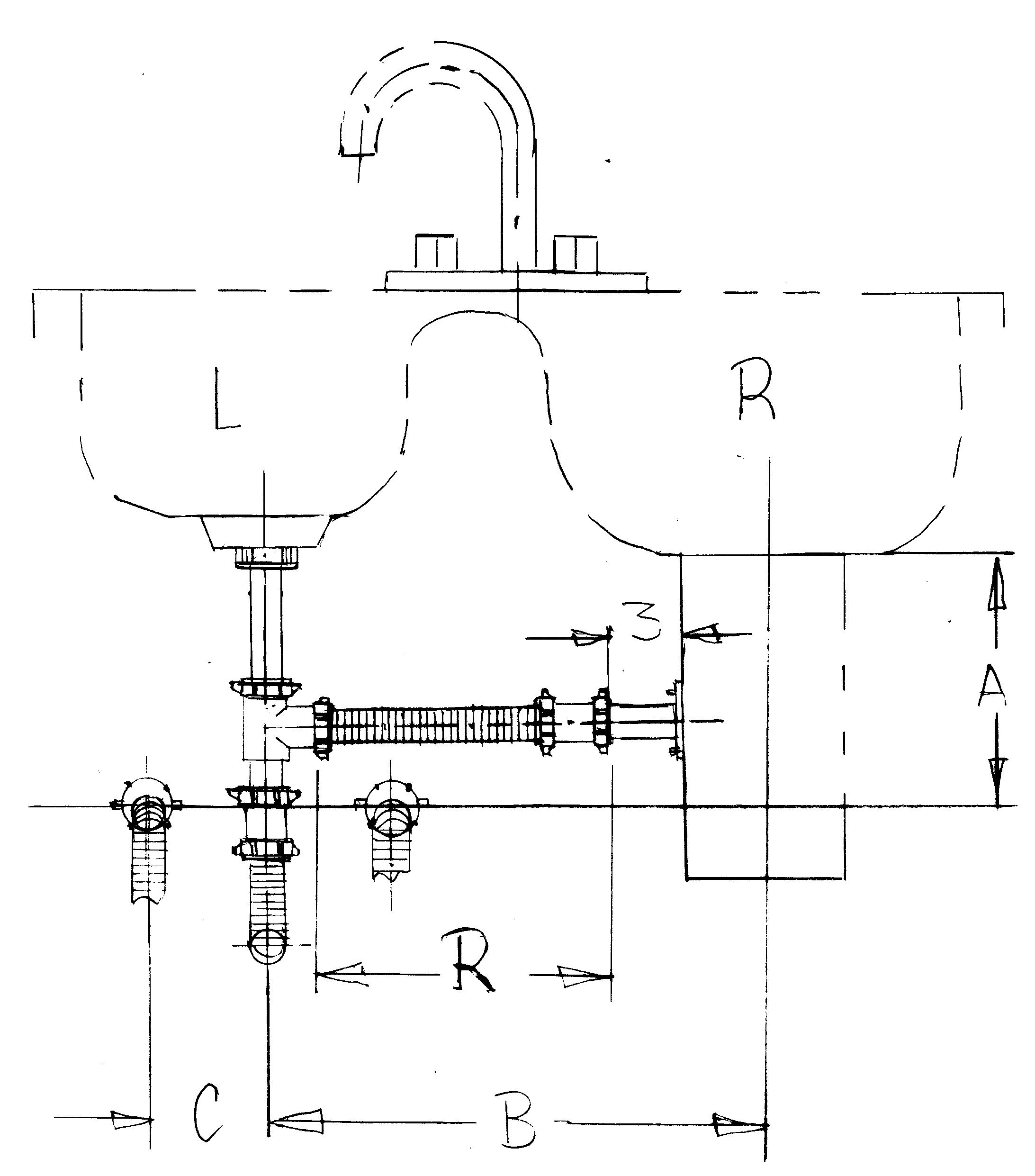Fig.21A (video)  L=24 inches or CP22 R=B-2 B=Bmax Fig.21B (video) L= 24 inches or CP22  R=B-4 B=Bmax

Flex p-trap

Full

Side view of Fig.21A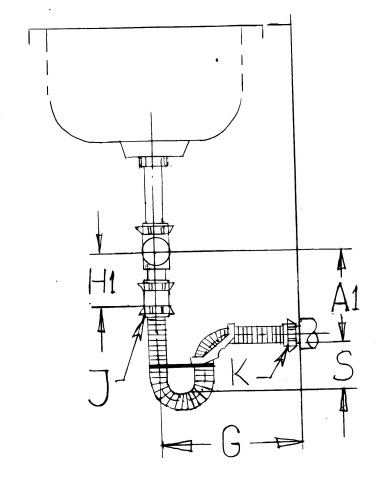Fig.22 (video)

G=8---10 inches, see the bathroom sink

G=10—14 inches

H1—length of tailpiece (end outlet tee)

A1—position of drain

L=length of flex p-trap

8 inches—length of U shape for two inches water seal (2+2+4)

2 inches—length of coupling

Minimum water seal of p-trap with adapter is 3.0 inches

E=0-4 inches—eccentricity (left or right)

S—water seal

Minimum water seal of the p-trap without adapter is 2.0 inches

For A1-H1=0  G=14

L=20 inches---Flex p-trap 2 inches water seal E=0 inches left, right

L=22 inches---Flex p-trap2-3 inches water seal E=2-0 inches left, right

Lmax=24 inches---Flex p-trap 3-4 inches water seal E=4-0 inches left, right

If you move the end of the p-trap right, left or down the water seal will decrease, but if you move it up the water seal will increase. For L=24 inches, it is recommended to have a minimum water seal of 2 inches without adapter, because the water and the adapter will increase the weight. Because of this, we recommend using the Connecting tube with p-trap (CP-trap) Fig.23.where G=4-14 inches. The adapter can be used over L=16 inches length.

Measurement

Our converter formula was specially designed to make you figure out your job a lot easier. Measure the distance JK in space and the length of Flex p-trap will be:

L=JK+10”

J—end of outlet tee

K—bottom of drain

CP-trap

Connecting tube with p-trap

Side view of Fig. 21B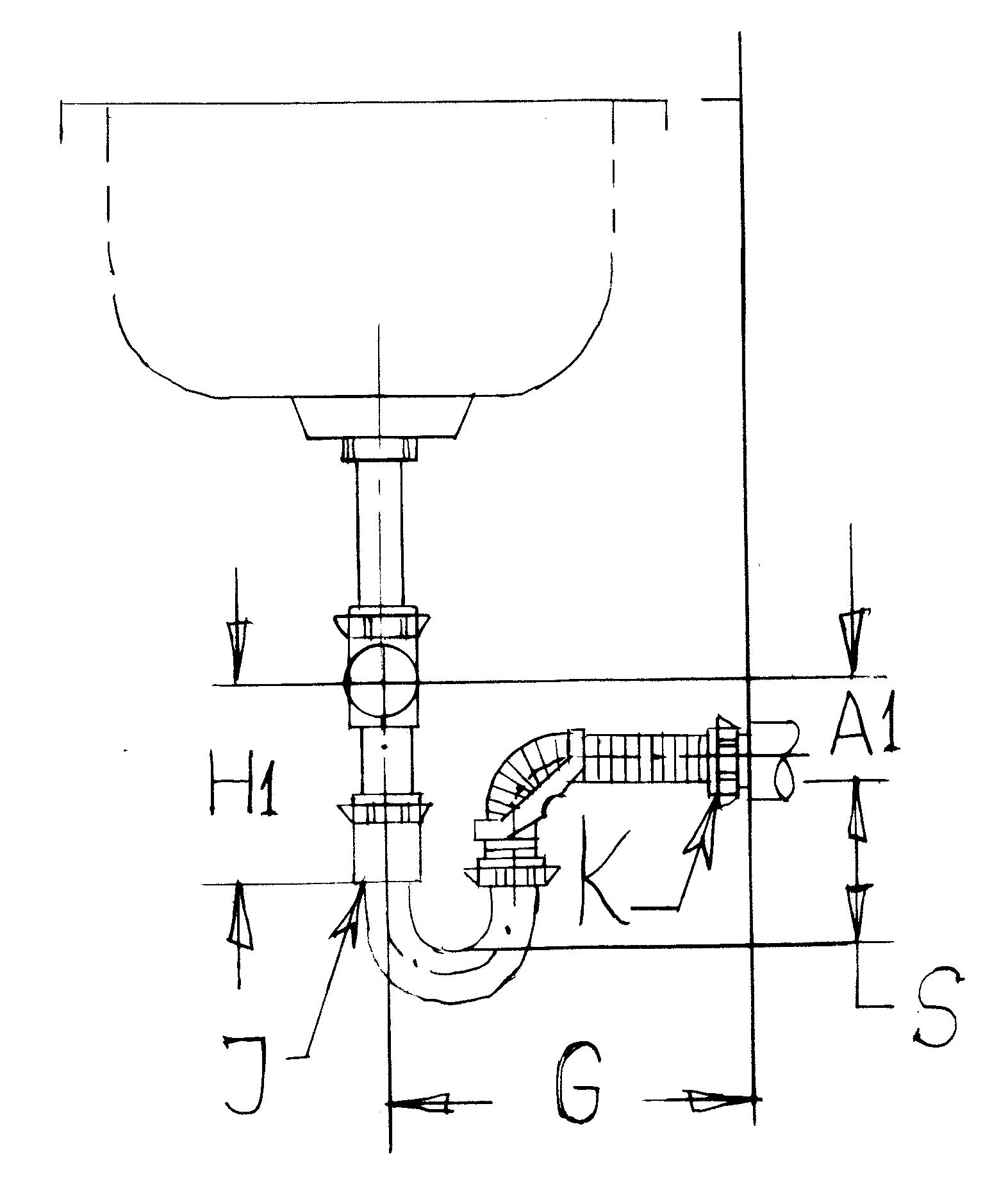Fig.23 (video 23A, video 23B)

G=4—10 inches, see the bathroom sink

G=10---14 inches E=0-4

L=CP---connecting tube wit p-trap

E--eccentricity

H1—length of tailpiece (end outlet tee)

A1—position of drain

8 inches—length of U shape of regular p-trap

H1-A1=2  (for assembling the adaptor with tube we need 2 inches)

For G=14 inches and E=0

L = G-4+2+8=G+6=14+6=20 inches

Rotate the p-trap around tailpiece G will be D and drain can be moved right or left E=0-4

L=D-4+2+8=D+6

L=D+6

For G=14    E=4      D2=E2+G2

D2=196+16    D=14.5

L=D+6=14.5+6=20.5=20

Rotate p-trap around tailpiece 180 degrees and E=4

L=D+4+2+8=D+14

L=D+14=14.5+14=28.5   Lmax=24

The p-trap with 24 inches length can’t be rotated full 180 degrees but will cover G=4-14 and E=0-4 inches.

For H1-A1=2  G=14

L=20 inches—Flex p-trap, 3 inches water seal E=0 inches left, right

L=22 inches—Flex p-trap, 3-4 inches water seal E=3-0 inches left, right

L=24 inches—Flex p-trap, 4-5 inches water seal E=4-0 inches left, right

Water seal S=3-5  E=0  G=14

Smin=3 water seal

Lmin=G+6=14+6=20 inches

If you add 2 inches to the length of p-trap, the water seal will increase with 1 inches

T=(S-3)2      T+Lmin=L   (S-3)2+20=L    2S-6+20=L   S=L/2-14/2   S=L/2-7

For T=0               L=20      E=0   0=2S-6  S=3”

T=2              L=22      E=0   S=22/2-7=4”

T=4              L=24      E=0   S=24/2-7=5”

If H1=constant,  G=14  E=0 and Smin=3 the drain can be lift up with 2 inches for Flex p-trap with L=22 inches and with 4 inches for Flex p-trap L=24 inches

The p-trap with 24 inches length can’t be rotated full 180 degrees but will cover G=4-14 and E=0-4 inches.

Measurement

Our converter formula was specially designed to make you figure out your job a lot easier. Measure distance JK in space and length of Flex p-trap will be:

L=JK+8”

J—end of outlet tee

K—bottom of drain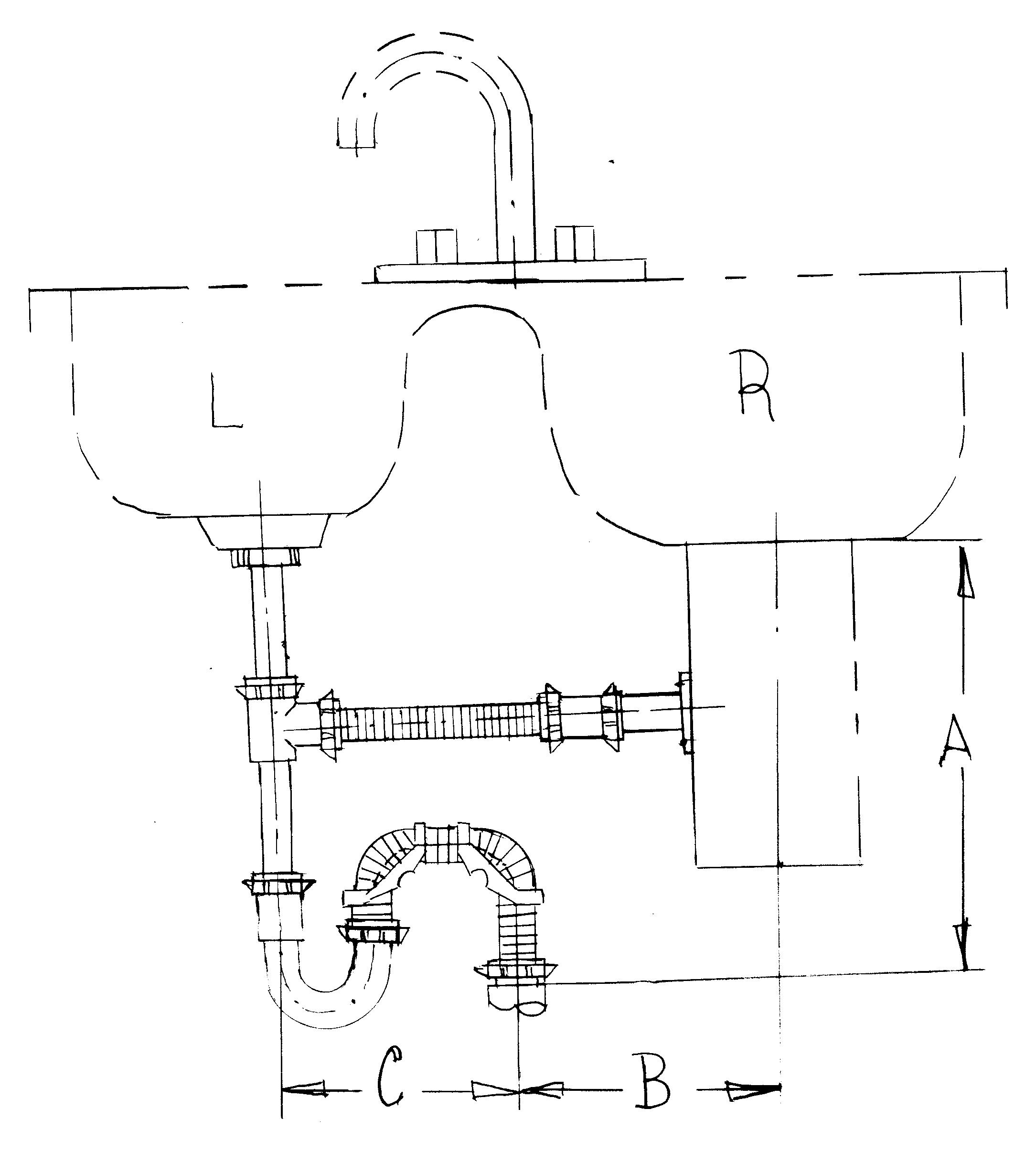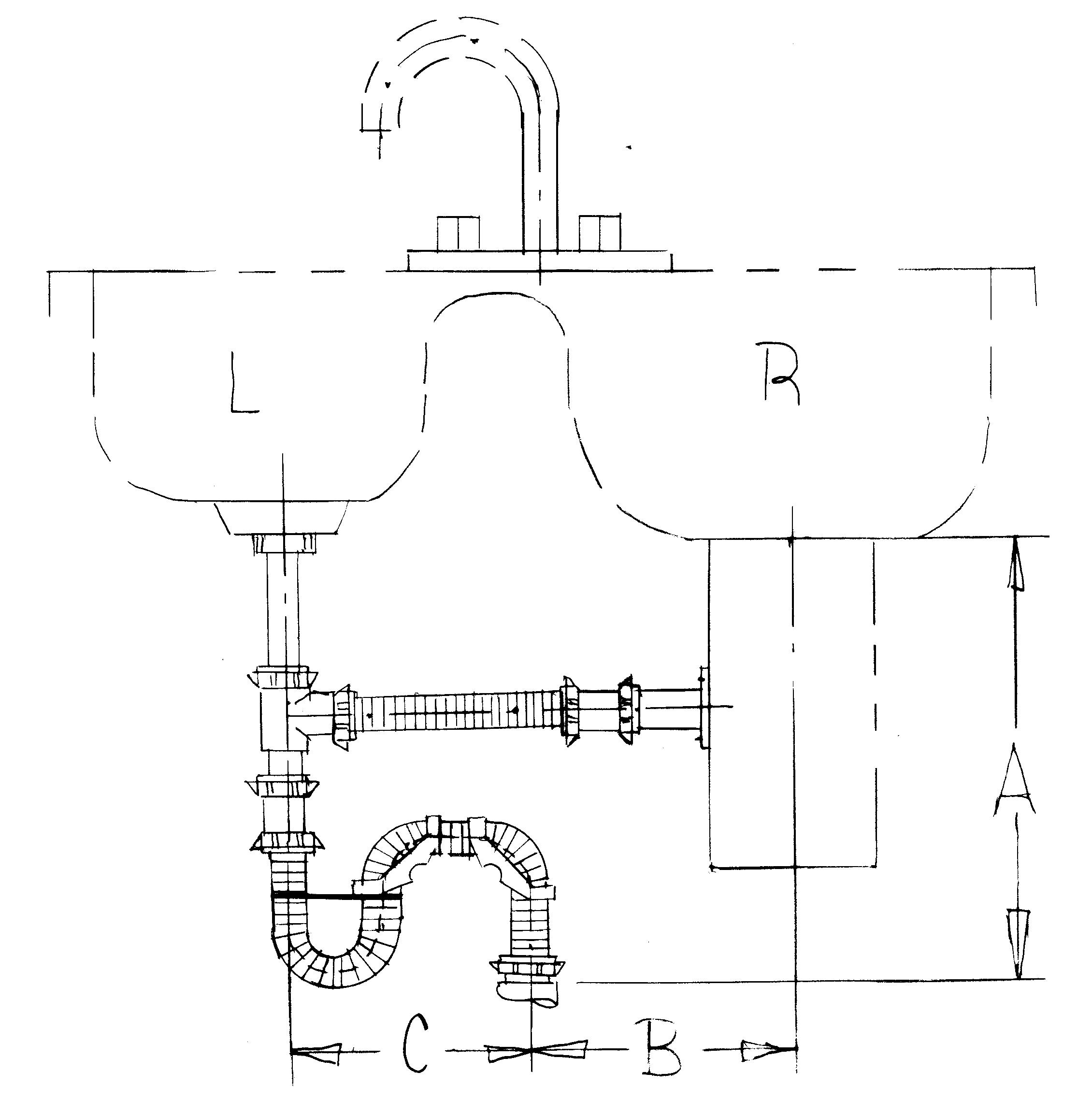Fig.24 (video) L=SP 24 see the bathroom sink R= Bmax-4    Bmax=B+C SP= s-trap with p-trap Fig.25 (video) L= S 24 see the bathroom sink R=Bmax-4 Bmax=B+C S=s-trap only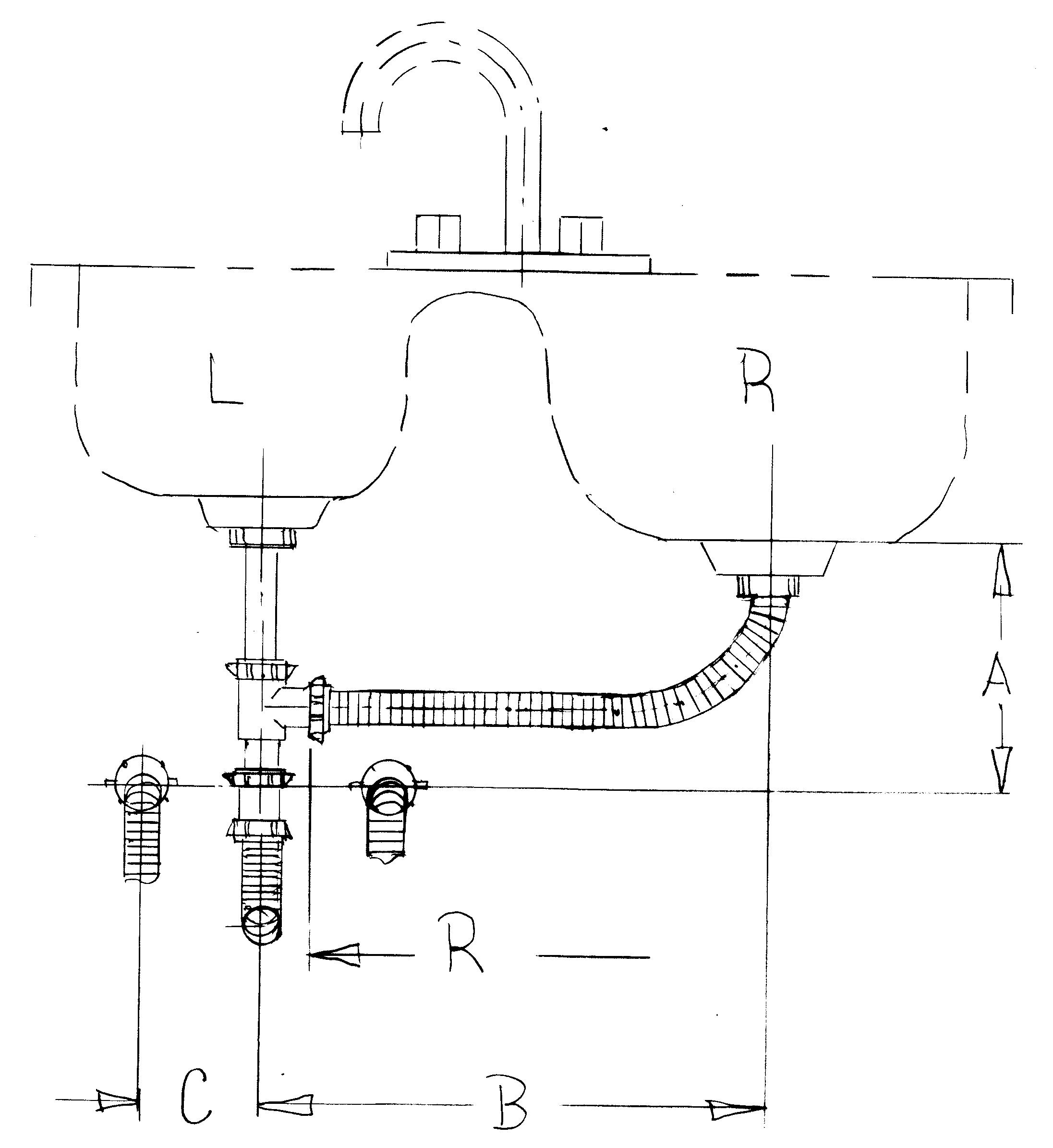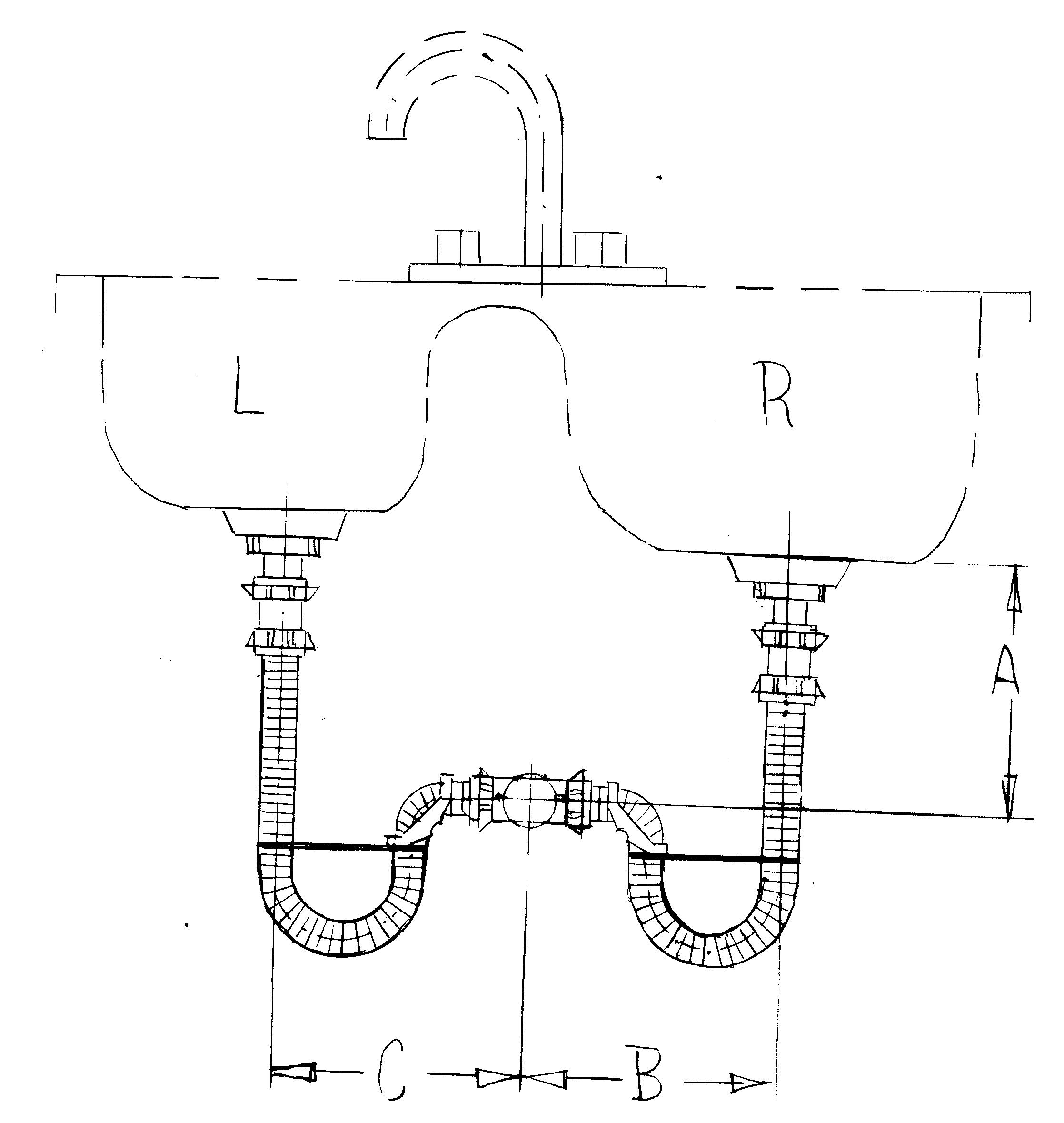Fig.26   L=24 inches or CP22  R=Bmax+2 Fig.27 L=24 inches and adapter R=24 inches and adapter